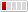3 Replies Latest reply on Oct 3, 2013 9:01 AM by Steven Hawkins

# SQL Translation errorHi,

I have a very long Oracle SQL which is being translated incorrectly by the jdbc translator and hence it runs into error. Although I was able to make a short query and re-produce the problem. Here it is:

Oracle Query (which works fine when executed in database):

SELECT T.X_ID,

T.X_ID_X,

DT_C7,

CASE

WHEN DT_C7 = '1'

THEN

(SELECT '1'

FROM XXX.XX_MV A

WHERE A.X_ID = T.X_ID AND ROWNUM < 2)

ELSE

''

END

AS DT_C7_ACTUAL

FROM XXX.ANALYSES T

WHERE X_ID_X IS NOT NULL

Teiid Designer Query (this was created after importing the Oracle objects and it compiles without error):

SELECT T.X_ID,

T.X_ID_X,

T.DT_C7,

CASE

WHEN T.DT_C7 = '1'

THEN

(SELECT '1'

FROM XXX.XX_MV AS A WHERE A.X_ID = T.X_ID LIMIT 1) ELSE '' END AS DT_C7_ACTUAL

FROM

XXX.ANALYSES AS T

WHERE

T.X_ID_X IS NOT NULL

But when the above query is executed I am getting the following error:

Error Code:904 Message:'ORA-00904: "G_0"."X_ID": invalid identifier

I checked the SQL generated by Teiid at runtime and it looks like the following:

SELECT v_0.c_0,

v_0.c_1,

v_0.c_2,

v_0.c_3

FROM (SELECT g_0.X_ID AS c_0,

g_0.X_ID_X AS c_1,

g_0.DT_C7 AS c_2,

CASE

WHEN g_0.DT_C7 = '1'

THEN

(SELECT c_0

FROM (SELECT '1' AS c_0

FROM "XXX"."XX_MV" g_1

WHERE g_1.X_ID = g_0.X_ID)

WHERE ROWNUM <= 1)

ELSE

''

END

AS c_3

FROM "XXX"."ANALYSES" g_0

WHERE     g_0.X_ID_X IS NOT NULL

The above query does not execute in Oracle and produces the same error as Teiid execution. I think the translator is not created the proper query.

Please let me know if this is a real problem and is we have a work around for this.

Appreciate any help!!

Regards

Tanmoy